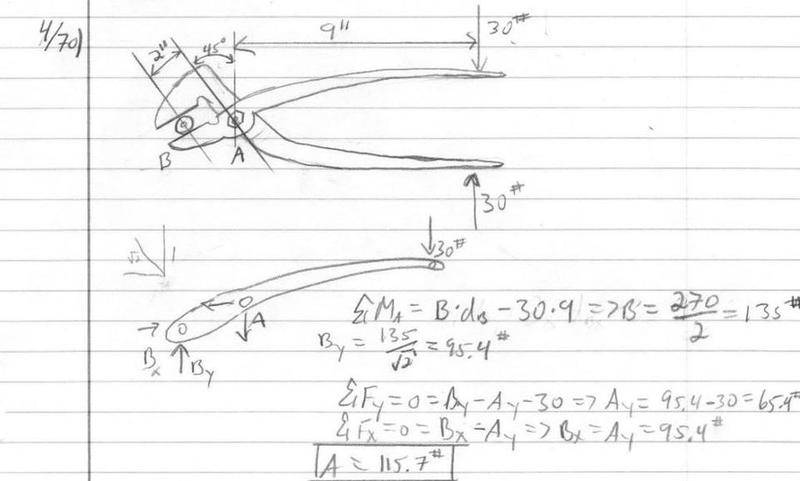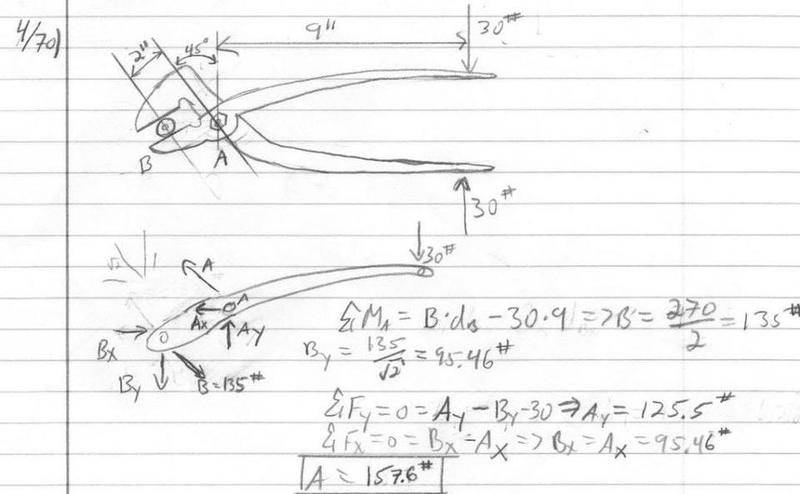# Forces on pin Did the problem but cant check answer

## Homework StatementWe are supposed to compute the force supported by pin at A for the slip joint pliers.
The book says they are under a 30# grip.
I summed forces about A to find B, then broke B up into components by dividing by $$\sqrt{2}$$ and then I summed forces for Ax and Ay and found A.

Let me know if you can't read part of my handwriting.

nvn
Homework Helper
frozenguy: That is currently incorrect. You used B in your calculation without showing the corresponding B vector on your free-body diagram. Did you try a summation of forces or moments on your answers, to see if they are in equilibrium? Keep trying.

Also, generally always maintain four significant digits throughout all your intermediate calculations, then round only the final answer to three significant digits, unless the first significant digit of the final answer is 1, in which case round the final answer to four significant digits.

Now I'm getting kind of confused. If I take moments around B, A should be able to balance out the 30lb moment. So shouldn't A balance out the 30 moment by it self? Thats what I dont get, because if I then take moments about A, B has to balance 30 all by itself. But in the real world, moments aren't dependent on which one you look at, what am I missing here?

nvn
Homework Helper
You can take moments about any point you wish; therefore, taking moments about point A is fine. Show vector B in your free-body diagram for your moment summation equation about point A.

So then B is 135lb at 45 deg above positive x axis.. So if I sum moments around C, I can find A?

I recommend you draw to scale the triangle of forces on this free body diagram. That should uncover your error.

nvn
Homework Helper
frozenguy: Why do you think force vector B is parallel to the jaw?

frozenguy: Why do you think force vector B is parallel to the jaw?

Because of the direction of the component forces, but looking at the picture, intuition says that b's force vector should be the other direction, perpendicular to the face of the jaw ya?

Oh, in my summation of Fx at the end, those are supposed to be Ax's

nvn
Homework Helper
frozenguy: That is correct; force vector B should be perpendicular to the jaw.

I'm not confident about my answer because of how I obtained B. While doing one problem in my book that had the answer displayed, I couldn't do what I did in this problem to get the magnitude of the vector. It like, had nothing to do with the angle of the member. The angle of the member was different then the angle the vector components gave me.

nvn
Homework Helper
Try it again, and we'll see if you get the right answer this time.